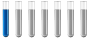Need help with analytical chemistry QuesAuthor Message

NewbieJoined: 29 Aug 2019
Posts: 1# Need help with analytical chemistry Ques

I am trying to solve this question but in vain if anyone can explain it will be great.

Q: Calculate the solubility of the solutes Th(OH)4 for solution in which the cation concentration is 0.050M.

I found the Ksp which is 1.1*10^-15 and i solved it this way :-

[Th+]+[4OH-]=1.1x10^-15
4[OH]= 4√1.0*10^-15 = 1.8x10^-4
1/4(1.8*10^-4)
=4.5x10^-5 = Final Ans

I have an exam and i am struck with this question pls tell me what is my mistake?Distinguished MemberJoined: 27 Sep 2017
Posts: 416
Location: Berlin, GermanyYou have mistypings. Kl = [Th4+] * [(OH-)^4] Th = x , OH- = 4x Kl = x* (4x)^4 Kl = 256 * x^5 x =5√ (Kl/256) x = 5√(1.1 * 10^-15/256) x = 3,36 * 10^-4 OH- = 4*x OH- = 1,34 * 10^-3 mol/lDisplay posts from previous: All Posts1 Day7 Days2 Weeks1 Month3 Months6 Months1 Year Oldest FirstNewest FirstPage 1 of 1